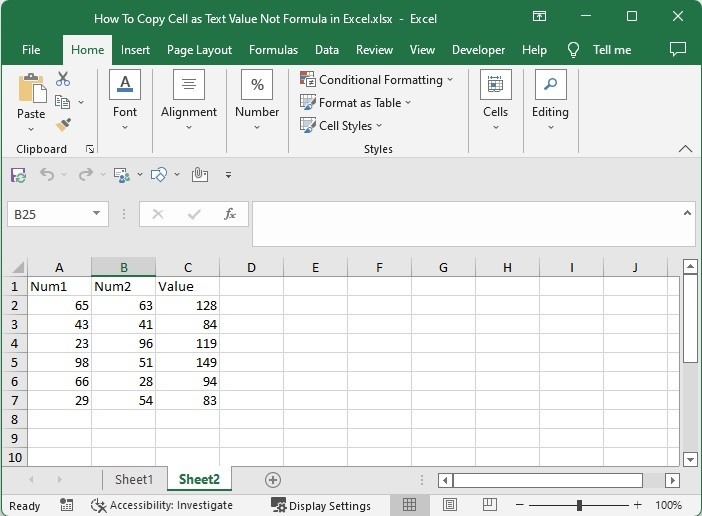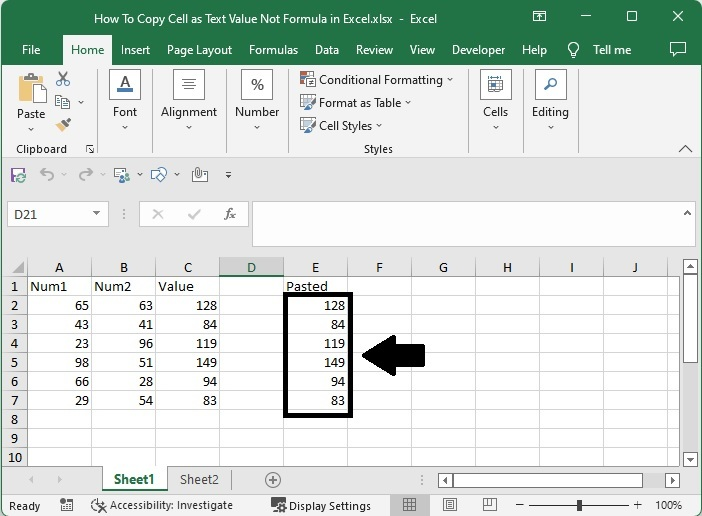# How to Copy Cell as Text Value Not Formula in Excel?

If we have formula cells on an Excel sheet and we try to copy them in the default way, the formula is copied instead of the values. This can create a mess if we try to copy the data from one sheet to another, as we also change the reference values in the sheet for the formula. Manually typing the cell valves into a new list of cells and then copying them is our direct solution. This can be a time-consuming and inaccurate process.

We can use a simple trick mentioned in this tutorial to complete our task in a faster and more accurate way. Read this article to learn how to copy a cell as a text value, not a formula, in Excel. We can finish the task by using Excel's paste‑special function.

Here, we will first copy the cells in the default way and then use the paste special operation while pasting the data. Let's go over a simple procedure for copying cells as text values rather than formulas in Excel.

### Step 1

Let us consider an Excel sheet where we have the list of formula cells along with their reference cells, similar to the below image.If we try to copy and paste the cells directly, then the values will return to an error as the reference of the formula needs to be changed, and the formula we use will be displayed in the formula box instead of a value.

### Step 2

For the special method, select the formula values you need to copy, use the command "CTRL + C" to copy the cells, click on the cell you want to start your list from, click on paste under home, and select paste as values as shown in the below image.

Select data > CTRL + C > Empty cell > Paste > Paste as values## Conclusion

In this tutorial, we used a simple example to demonstrate how we can copy cells as text values, not formulas, in Excel.